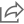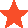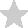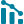Casio fx-115ES PLUS Natural-V.P.A.M. 2nd Edition Scientific Calculator, White, FX-115ESPLS2-S
This product ad may change over timeShare
(36)

# Casio fx-115ES PLUS Natural-V.P.A.M. 2nd Edition Scientific Calculator, White, FX-115ESPLS2-S

Make the Casio fx-115ES PLUS Scientific Calculator your high school or college companion. With 460 total functions, this calculator is ideal for students in general math, pre-algebra, algebra I and II, geometry, trigonometry, statistics, calculus, chemistry and engineering. 2.5" N…
Make the Casio fx-115ES PLUS Scientific Calculator your high school or college companion. With 460 total functions, this calculator is ideal for students in general math, pre-algebra, algebra I and II, geometry, trigonometry, statistics, calculus, chemistry and engineering. 2.5" Natural Textbook Display shows up to 4 lines depending on the function. Formulas and results are presented exactly as they would appear in a textbook. 460 functions are included, such as prime factorization, hyperbolic functions, greatest common divisor and least common multiple, summation notation and verify computations. Matrix mode allows you to perform matrix operations on matrices with up to 3 rows and 3 columns, enter vectors and perform vector calculations. Simultaneous and polynomial solver helps you compute simultaneous linear equations with 2 or 3 unknowns, quadratic equations and cubic equations. Fraction calculations include simplifying fractions and roots, recurring decimal display, remainder calculation and integer division with remainder. Calculate linear regression, quadratic regression, logarithmic regression, e exponential regression, ab exponential regression, power regression and inverse regression. Complex number calculations include addition, subtraction, multiplication, division, augment and absolute value, reciprocal, square and cube and conjugate complex numbers. Converts decimals to fractions and mixed numbers. Also converts metric measurements. Random integer generator aids in activities. Backed by the manufacturer's 1-year limited warranty. Renewably powered - designed to work without relying on an electric connection or disposable batteries, potentially helping you save money.
See more
\$19.99
Visit Office Depot

## Price History

Something went wrong. Please try again later.

## User Reviews

Recent reviews
FeaturesJan 23, 2022
I have been using it for few months and its working great. Super easy operation and efficient Full review by officedepot.comJan 18, 2022
I bought this calculator to replace another one and I love all of the extra features. This calculator converts from fractions to decimals. Full review by officedepot.comMay 4, 2022
It is true that the Casio fx-115ES Plus, 2nd edition is powerful and has many functions, but the construction of the calculator itself is the cheapest plastic. It is very light and flimsy, like it ca… Full review by walmart.com

## Description

Make the Casio fx-115ES PLUS Scientific Calculator your high school or college companion. With 460 total functions, this calculator is ideal for students in general math, pre-algebra, algebra I and II, geometry, trigonometry, statistics, calculus, chemistry and engineering. 2.5" Natural Textbook Display shows up to 4 lines depending on the function. Formulas and results are presented exactly as they would appear in a textbook. 460 functions are included, such as prime factorization, hyperbolic functions, greatest common divisor and least common multiple, summation notation and verify computations. Matrix mode allows you to perform matrix operations on matrices with up to 3 rows and 3 columns, enter vectors and perform vector calculations. Simultaneous and polynomial solver helps you compute simultaneous linear equations with 2 or 3 unknowns, quadratic equations and cubic equations. Fraction calculations include simplifying fractions and roots, recurring decimal display, remainder calculation and integer division with remainder. Calculate linear regression, quadratic regression, logarithmic regression, e exponential regression, ab exponential regression, power regression and inverse regression. Complex number calculations include addition, subtraction, multiplication, division, augment and absolute value, reciprocal, square and cube and conjugate complex numbers. Converts decimals to fractions and mixed numbers. Also converts metric measurements. Random integer generator aids in activities. Backed by the manufacturer's 1-year limited warranty. Renewably powered - designed to work without relying on an electric connection or disposable batteries, potentially helping you save money.

## Price History

Something went wrong. Please try again later.Tracking price
We added this to your collection and will notify you if the price dropsView collection
Price tracking canceled
We removed this from your collectionView collection
Something went wrong. Please try again later.
Track price